# Modeling and correcting fragment sequence bias

Here we show a brief example of using the alpine package to model bias parameters and then using those parameters to estimate transcript abundance. We load a metadata table and a subset of reads from four samples from the GEUVADIS project. For more details on these files, see `?alpineData` in the alpineData package.

``````library(alpineData)
dir <- system.file("extdata",package="alpineData")
stringsAsFactors=FALSE)
``````
``````##       Title Performer   Date Population
## 1 ERR188297     UNIGE 111124        TSI
## 2 ERR188088     UNIGE 111124        TSI
## 3 ERR188204  CNAG_CRG 111215        TSI
## 4 ERR188317  CNAG_CRG 111215        TSI
``````

A subset of the reads from one of the samples:

``````library(GenomicAlignments)
ERR188297()
``````
``````## GAlignmentPairs object with 25531 pairs, strandMode=1, and 0 metadata columns:
##           seqnames strand   :              ranges  --              ranges
##              <Rle>  <Rle>   :           <IRanges>  --           <IRanges>
##               1      +   : 108560389-108560463  -- 108560454-108560528
##               1      -   : 108560454-108560528  -- 108560383-108560457
##               1      +   : 108560534-108600608  -- 108600626-108606236
##               1      -   : 108569920-108569994  -- 108569825-108569899
##               1      -   : 108587954-108588028  -- 108587881-108587955
##       ...      ...    ... ...                 ... ...                 ...
##           X      +   : 119790596-119790670  -- 119790717-119790791
##           X      +   : 119790988-119791062  -- 119791086-119791160
##           X      +   : 119791037-119791111  -- 119791142-119791216
##           X      +   : 119791348-119791422  -- 119791475-119791549
##           X      +   : 119791376-119791450  -- 119791481-119791555
##   -------
##   seqinfo: 194 sequences from an unspecified genome
``````

Before we start, we need to write these paired-end reads, here stored in a R/Bioconductor data object, out to a BAM file, because the alpine software works with alignments stored as BAM files. This is not a typical step, as you would normally have BAM files already on disk. We write out four BAM files for each of the four samples contained in alpineData. So you can ignore the following code chunk if you are working with your own BAM files.

``````library(rtracklayer)
dir <- tempdir()
# the reads are accessed with functions named
# after the sample name. the following line calls
# the function with the sample name and saves
gap <- match.fun(sample.name)()
file.name <- file.path(dir,paste0(sample.name,".bam"))
export(gap, con=file.name)
}
stopifnot(all(file.exists(bam.files)))
``````

Now we continue with the typical steps in an alpine workflow. To fit the bias model, we need to identify single-isoform genes. We used the following chunk of code (here not evaluated) to generate a GRangesList of exons per single-isoform gene.

``````library(ensembldb)
gtf.file <- "Homo_sapiens.GRCh38.84.gtf"
txdb <- EnsDb(gtf.file) # already an EnsDb
txdf <- transcripts(txdb, return.type="DataFrame")
tab <- table(txdf\$gene_id)
one.iso.genes <- names(tab)[tab == 1]
# pre-selected genes based on medium to high counts
selected.genes <- scan("selected.genes.txt", what="char")
one.iso.txs <- txdf\$tx_id[txdf\$gene_id %in%
intersect(one.iso.genes, selected.genes)]
ebt0 <- exonsBy(txdb, by="tx")
ebt.fit <- ebt0[one.iso.txs]
``````

Here we pick a subset of single-isoform genes based on the number of exons, and the length. We show in comments the recommended parameters to use in selecting this subset of genes, although here we use different parameters to ensure the building of the vignette takes only a short period of time and does not use much memory.

``````library(GenomicRanges)
``````
``````library(alpine)
data(preprocessedData)
# filter small genes and long genes
min.bp <- 600
max.bp <- 7000
gene.lengths <- sum(width(ebt.fit))
summary(gene.lengths)
``````
``````##    Min. 1st Qu.  Median    Mean 3rd Qu.    Max.
##    66.0   689.8  1585.0  2147.0  3352.5  6103.0
``````
``````ebt.fit <- ebt.fit[gene.lengths > min.bp & gene.lengths < max.bp]
length(ebt.fit)
``````
``````##  25
``````
``````set.seed(1)
# better to use ~100 genes
ebt.fit <- ebt.fit[sample(length(ebt.fit),10)]
``````

## Defining a set of fragment types

Robust fitting of these bias parameters is best with ~100 medium to high count genes, e.g. mean count across samples between 200 and 10,000. These counts can be identified by featureCounts from the Rsubread Bioconductor package, for example. It is required to specify a minimum and maximum fragment size which should be lower and upper quantiles of the fragment length distribution. The `minsize` and `maxsize` arguments are recommended to be roughly the 2.5% and 97.5% of the fragment length distribution. This can be quickly estimated using the helper function getFragmentWidths, iterating over a few single-isoform genes with sufficient counts:

``````w <- getFragmentWidths(bam.files, ebt.fit[])
c(summary(w), Number=length(w))
``````
``````##      Min.   1st Qu.    Median      Mean   3rd Qu.      Max.    Number
##   79.0000  161.0000  188.0000  196.8846  224.0000  468.0000 1768.0000
``````
``````quantile(w, c(.025, .975))
``````
``````##   2.5%  97.5%
## 121.00 321.65
``````

It is also required to specify the read length. Currently alpine only supports unstranded, paired-end RNA-seq with fixed read length. Differences of +/- 1 bp in read length across samples can be ignored.

``````getReadLength(bam.files)
``````
``````## ERR188297 ERR188088 ERR188204 ERR188317
##        75        75        76        76
``````

Here we use a very limited range of fragment lengths for speed, but for a real analysis we would suggest using the minimum and maximum of the quantiles computed above across all samples (the minimum of the lower quantiles and the maximum of the upper quantiles).

``````library(alpine)
library(BSgenome.Hsapiens.NCBI.GRCh38)
minsize <- 125 # better 80 for this data
maxsize <- 175 # better 350 for this data
gene.names <- names(ebt.fit)
names(gene.names) <- gene.names
``````

The following function builds a list of DataFrames which store information about the fragment types from each gene in our training set.

``````system.time({
fragtypes <- lapply(gene.names, function(gene.name) {
buildFragtypes(exons=ebt.fit[[gene.name]],
genome=Hsapiens,
minsize=minsize,
maxsize=maxsize,
gc.str=FALSE)
})
})
``````
``````##    user  system elapsed
## 141.216   0.976 143.510
``````
``````print(object.size(fragtypes), units="auto")
``````
``````## 99.7 Mb
``````

We can examine the information for a single gene:

``````head(fragtypes[], 3)
``````
``````## DataFrame with 3 rows and 14 columns
##       start       end             relpos   fraglen        id fivep.test
##   <integer> <integer>          <numeric> <integer> <IRanges>  <logical>
## 1         1       125  0.010322792069474       125     1-125      FALSE
## 2         1       126  0.010322792069474       126     1-126      FALSE
## 3         1       127 0.0104866459118466       127     1-127      FALSE
##            fivep threep.test                threep                gc
##   <DNAStringSet>   <logical>        <DNAStringSet>         <numeric>
## 1  ATCCGGGGCAGCG        TRUE TCAATTCAAAATGTCTCAGGA             0.768
## 2  ATCCGGGGCAGCG        TRUE GTCAATTCAAAATGTCTCAGG 0.761904761904762
## 3  ATCCGGGGCAGCG        TRUE TGTCAATTCAAAATGTCTCAG 0.763779527559055
##   <integer> <integer> <integer>   <integer>
## 1  32277664  32309735  32277738    32277714
## 2  32277664  32309736  32277738    32277715
## 3  32277664  32309737  32277738    32277716
``````

## Defining and fitting bias models

The definition of bias models is extremely flexible in alpine. The `models` argument should be given as a list, where each element is model. The model itself should be provided as a list with elements `formula` and `offset`. Either `formula` or `offset` can be set to `NULL` for a given model. The allowable offsets are `fraglen` and/or `vlmm` which should be provided in a character vector. Offsets are only estimated once for all models, so setting `formula=NULL` only makes sense if extra offsets are desired which were not already calculated by other models.

Any kind of R formula can be provided to `formula`, making use of the fragment features:

• `gc` (fragment GC content from 0 to 1)
• `relpos` (fragment midpoint relative position from 0 to 1)
• `GC40.80`, `GC40.90`, `GC20.80`, `GC20.90` (indicator variables indicating the presence of, e.g. a 40 bp stretch of 80% or higher GC content within the fragment)

These fragment features reference columns of information stored in `fragtypes`. Interactions between these terms and offsets are also possible, e.g. `gc:fraglen`.

Note: It is required to provide formula as character strings, which are converted internally into formula, due to details in how R formula make copies of objects from the environment.

``````models <- list(
"GC" = list(
formula = "count ~ ns(gc,knots=gc.knots,Boundary.knots=gc.bk) + ns(relpos,knots=relpos.knots,Boundary.knots=relpos.bk) + gene",
offset=c("fraglen")
),
"all" = list(
formula = "count ~ ns(gc,knots=gc.knots,Boundary.knots=gc.bk) + ns(relpos,knots=relpos.knots,Boundary.knots=relpos.bk) + gene",
offset=c("fraglen","vlmm")
)
)
``````

Here we fit one bias model, `GC`, using fragment length, fragment GC content, relative position, and a term for differences in expression across the genes (`+ gene`).

We fit another bias model, `all`, with all the terms of the first but additionally with read start bias (encoded by a Variable Length Markov Model, or VLMM).

Note: It is required if a formula is provided that it end with ```+ gene``` to account for differences in base expression levels while fitting the bias parameters.

The knots and boundary knots for GC content (`gc`) and relative position (`relpos`) splines have reasonable default values, but they can be customized using arguments to the fitBiasModels function.

The returned object, `fitpar`, stores the information as a list of fitted parameters across samples.

``````system.time({
fitpar <- lapply(bam.files, function(bf) {
fitBiasModels(genes=ebt.fit,
bam.file=bf,
fragtypes=fragtypes,
genome=Hsapiens,
models=models,
minsize=minsize,
maxsize=maxsize)
})
})
``````
``````##    user  system elapsed
## 308.224   1.724 310.163
``````
``````# this object saved was 'fitpar.small' for examples in alpine man pages
# fitpar.small <- fitpar
``````

## Visually exploring the bias parameters

Note that with more basepairs between `minsize` and `maxsize` and with more genes used for estimation, the bias parameters would be more precise. As estimated here, the curves look a bit wobbly. Compare to the curves that are fit in the alpine paper (see `citation("alpine")`). The estimated spline coefficients have high variance from too few observations (paired-end fragments) across too few genes.

First we set a palette to distinguish between samples

``````library(RColorBrewer)
palette(brewer.pal(8,"Dark2"))
``````

The fragment length distribution:

``````perf <- as.integer(factor(metadata\$Performer))
plotFragLen(fitpar, col=perf)
``````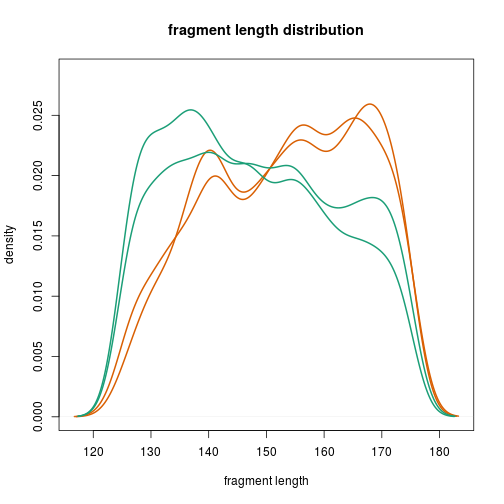The fragment GC bias curves:

``````plotGC(fitpar, model="all", col=perf)
``````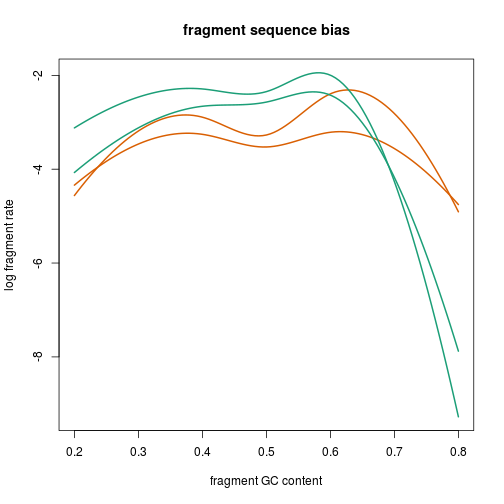The relative position curves:

``````plotRelPos(fitpar, model="all", col=perf)
``````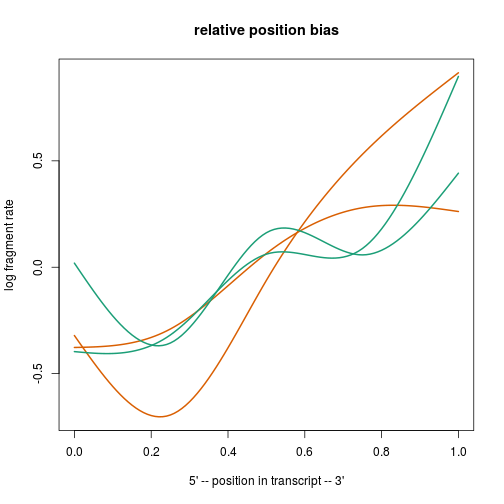A 0-order version of the VLMM (note that the VLMM that is used in the model includes positions that are 1- and 2-order, so this plot does not represent the final VLMM used in bias estimation or in estimation of abundances).

``````plotOrder0(fitpar[["ERR188297"]][["vlmm.fivep"]][["order0"]])
``````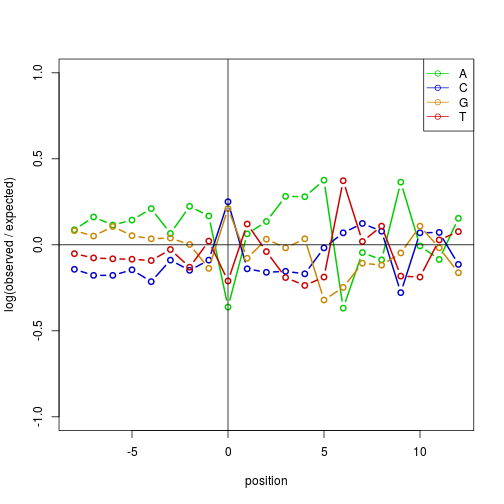``````plotOrder0(fitpar[["ERR188297"]][["vlmm.threep"]][["order0"]])
``````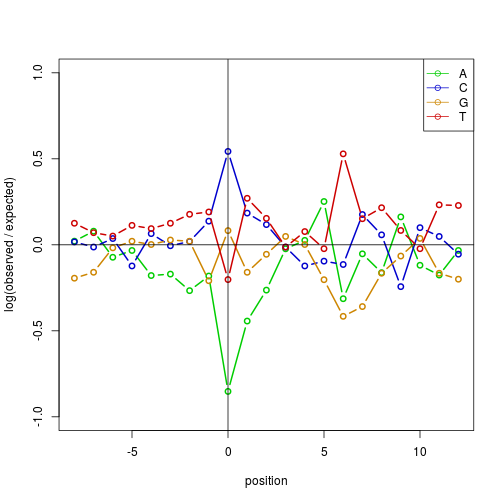A coefficient table for the terms in `formula`:

``````print(head(fitpar[["ERR188297"]][["summary"]][["all"]]), row.names=FALSE)
``````
``````##    Estimate Std. Error z value Pr(>|z|)
##  -6.9471098  1.3128651 -5.2916 1.21e-07
##   3.4507666  1.2635769  2.7310 6.32e-03
##   3.0326201  0.8803969  3.4446 5.72e-04
##   5.6733501  2.5740338  2.2041 2.75e-02
##  -3.6914944  2.0668845 -1.7860 7.41e-02
##   0.4844757  0.1618429  2.9935 2.76e-03
``````

## Estimating transcript abundances

We pick a subset of genes for estimating transcript abundances. If the gene annotation includes genes with transcripts which span multiple chromosomes or which do not have any overlap and are very far apart, splitGenesAcrossChroms and splitLongGenes, respectively, can be used to split these. For again merging any overlapping transcripts into “genes”, the mergeGenes function can be used. Here we use the ENSEMBL gene annotation as is.

The following code chunk is not evaluated but was used to select a few genes for demonstrating estimateAbundance:

``````one.iso.genes <- intersect(names(tab)[tab == 1], selected.genes)
two.iso.genes <- intersect(names(tab)[tab == 2], selected.genes)
three.iso.genes <- intersect(names(tab)[tab == 3], selected.genes)
set.seed(1)
genes.theta <- c(sample(one.iso.genes, 2),
sample(two.iso.genes, 2),
sample(three.iso.genes, 2))
txdf.theta <- txdf[txdf\$gene_id %in% genes.theta,]
ebt.theta <- ebt0[txdf.theta\$tx_id]
``````

Next we specify the set of models we want to use, referring back by name to the models that were fit in the previous step. Additionally, we can include any of the following models: `null`, `fraglen`, `vlmm`, or `fraglen.vlmm` which are the four models that can be fit using only offsets (none, either or both of the offsets).

``````model.names <- c("null","fraglen.vlmm","GC")
``````

Here we estimate FPKM-scale abundances for multiple genes and multiple samples. If `lib.sizes` is not specified, a default value of 1e6 is used. estimateAbundance works one gene at a time, where the `transcripts` argument expects a GRangesList of the exons for each transcript (multiple if the gene has multiple isoforms).

``````system.time({
res <- lapply(genes.theta, function(gene.name) {
txs <- txdf.theta\$tx_id[txdf.theta\$gene_id == gene.name]
estimateAbundance(transcripts=ebt.theta[txs],
bam.files=bam.files,
fitpar=fitpar,
genome=Hsapiens,
model.names=model.names)
})
})
``````
``````##     user   system  elapsed
##  803.380    4.140 1210.156
``````

Each element of this list has the abundances (`theta`) and average bias (`lambda`) for a single gene across all samples, all models, and all isoforms of the gene:

``````res[][["ERR188297"]][["GC"]]
``````
``````## \$theta
## ENST00000259030
##       0.3138475
##
## \$lambda
## ENST00000259030
##        80.04006
``````
``````res[][["ERR188297"]][["GC"]]
``````
``````## \$theta
## ENST00000477403 ENST00000468844 ENST00000361575
##     7.039680305     0.007816314     2.963646821
##
## \$lambda
## ENST00000477403 ENST00000468844 ENST00000361575
##        89.86951        77.09618        89.62648
``````

The extractAlpine function can be used to collate estimates from across all genes. extractAlpine will scale the estimates such that the total bias observed over all transcripts is centered at 1. The estimates produce by estimateAbundance presume a default library size of 1e6, but will be rescaled using the total number of fragments across genes when using extractAlpine (if this library size rescaling is not desired, choose `divide.out=FALSE`).

``````mat <- extractAlpine(res, model="GC")
mat
``````
``````##                    ERR188297   ERR188088   ERR188204    ERR188317
## ENST00000259030 5.561224e+03  17792.3723  10388.0341 1.308873e+04
## ENST00000304788 4.292888e+03   5554.4300   8512.5682 7.641947e+03
## ENST00000295025 1.335355e+04   9580.3667  18512.2160 1.530878e+04
## ENST00000394479 4.886171e+03   1108.4860   6363.7757 4.456278e+03
## ENST00000330871 1.532581e+04  33736.0980  10833.8633 1.156186e+04
## ENST00000587578 0.000000e+00      0.0000      0.0000 0.000000e+00
## ENST00000264254 3.860413e+04  49953.6885  55809.8417 6.374151e+04
## ENST00000416255 1.969685e+03   7840.6398   4798.5543 2.319688e+03
## ENST00000450127 4.075259e-32   1696.1784    565.4501 2.799949e-28
## ENST00000477403 1.247397e+05 129791.0327 236095.2131 1.603998e+05
## ENST00000468844 1.385013e+02    635.0712   1172.3366 1.154142e+03
## ENST00000361575 5.251437e+04  62200.9231  85026.3527 1.873892e+05
``````

If we provide a GRangesList which contains the exons for each transcript, the returned object will be a SummarizedExperiment. The GRangesList provided to `transcripts` does not have to be in the correct order, the transcripts will be extracted by name to match the rows of the FPKM matrix.

``````se <- extractAlpine(res, model="GC", transcripts=ebt.theta)
se
``````
``````## class: RangedSummarizedExperiment
## dim: 12 4
## assays(1): FPKM
## rownames(12): ENST00000259030 ENST00000304788 ... ENST00000468844
##   ENST00000361575
## rowData names(0):
## colnames(4): ERR188297 ERR188088 ERR188204 ERR188317
## colData names(0):
``````

The matrix of FPKM values can be scaled using the median ratio method of DESeq with the normalizeDESeq function. This is a robust method which removes systematic differences in values across samples, and is more appropriate than using the total count which is sensitive to very large abundance estimates for a minority of transcripts.

``````norm.mat <- normalizeDESeq(mat, cutoff=0.1)
``````

## Simulating RNA-seq data with empirical GC bias

The fragment GC bias which alpine estimates can be used in downstream simulations, for example in the polyester Bioconductor package. All we need to do is to run the plotGC function, but specifying that instead of a plot, we want to return a matrix of probabilities for each percentile of fragment GC content. This matrix can be provided to the `frag_GC_bias` argument of simulate_experiment.

We load a `fitpar` object that was run with the fragment length range [80,350] bp.

``````data(preprocessedData)
prob.mat <- plotGC(fitpar, "all", return.type=2)
``````
``````##       ERR188297 ERR188088  ERR188204  ERR188317
## 0    0.04366855 0.2561645 0.06914584 0.07234787
## 0.01 0.04936226 0.2725952 0.07667866 0.08005226
## 0.02 0.05578805 0.2900464 0.08501986 0.08856473
## 0.03 0.06302707 0.3085437 0.09424127 0.09795502
## 0.04 0.07116603 0.3281071 0.10441771 0.10829555
## 0.05 0.08029675 0.3487502 0.11562636 0.11966079
``````

If `return.type=0` (the default) the function makes the plot of log fragment rate over fragment GC content. If `return.type=1` the function returns the matrix of log fragment rate over percentiles of fragment GC content, and if `return.type=2`, the matrix returns probabilities of observing fragments based on percentiles of fragment GC content (the log fragment rate exponentiated and scaled to have a maximum of 1). The matrix returned by `return.type=2` is appropriate for downstream use with polyester.

## Plotting predicted fragment coverage

In the alpine paper, it was shown that models incorporating fragment GC bias can be a better predictor of test set RNA-seq fragment coverage, compared to models incorporating read start bias. Here we show how to predict fragment coverage for a single-isoform gene using a variety of fitted bias models. As with estimateAbundace, the model names need to refer back to models fit using fitBiasModels.

``````model.names <- c("fraglen","fraglen.vlmm","GC","all")
``````

The following function computes the predicted coverage for one single-isoform gene. We load a `fitpar` object that was run with the fragment length range [80,350] bp.

``````fitpar[][["model.params"]][c("minsize","maxsize")]
``````
``````## \$minsize
##  80
##
## \$maxsize
##  350
``````
``````system.time({
pred.cov <- predictCoverage(gene=ebt.fit[["ENST00000245479"]],
bam.files=bam.files["ERR188204"],
fitpar=fitpar,
genome=Hsapiens,
model.names=model.names)
})
``````
``````##    user  system elapsed
## 308.652   1.236 310.161
``````

We can plot the observed and predicted coverage for one of the genes:

``````palette(brewer.pal(9, "Set1"))
frag.cov <- pred.cov[["ERR188204"]][["frag.cov"]]
plot(frag.cov, type="l", lwd=3, ylim=c(0,max(frag.cov)*1.5))
for (i in seq_along(model.names)) {
m <- model.names[i]
pred <- pred.cov[["ERR188204"]][["pred.cov"]][[m]]
lines(pred, col=i, lwd=3)
}
legend("topright", legend=c("observed",model.names),
col=c("black",seq_along(model.names)), lwd=3)
``````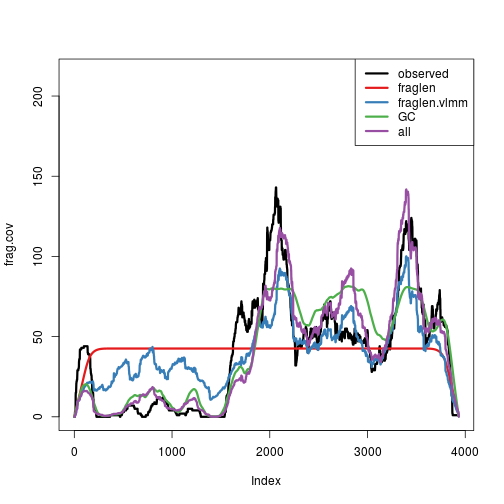## Session information

``````sessionInfo()
``````
``````## R version 3.5.1 Patched (2018-07-12 r74967)
## Platform: x86_64-pc-linux-gnu (64-bit)
## Running under: Ubuntu 16.04.5 LTS
##
## Matrix products: default
## BLAS: /home/biocbuild/bbs-3.8-bioc/R/lib/libRblas.so
## LAPACK: /home/biocbuild/bbs-3.8-bioc/R/lib/libRlapack.so
##
## locale:
##   LC_CTYPE=en_US.UTF-8       LC_NUMERIC=C
##   LC_TIME=en_US.UTF-8        LC_COLLATE=C
##   LC_MONETARY=en_US.UTF-8    LC_MESSAGES=en_US.UTF-8
##   LC_PAPER=en_US.UTF-8       LC_NAME=C
##  LC_MEASUREMENT=en_US.UTF-8 LC_IDENTIFICATION=C
##
## attached base packages:
##  stats4    parallel  stats     graphics  grDevices utils     datasets
##  methods   base
##
## other attached packages:
##   RColorBrewer_1.1-2
##   BSgenome.Hsapiens.NCBI.GRCh38_1.3.1000
##   BSgenome_1.50.0
##   alpine_1.8.0
##   rtracklayer_1.42.0
##   alpineData_1.7.1
##   GenomicAlignments_1.18.0
##   Rsamtools_1.34.0
##   Biostrings_2.50.0
##  XVector_0.22.0
##  SummarizedExperiment_1.12.0
##  DelayedArray_0.8.0
##  BiocParallel_1.16.0
##  matrixStats_0.54.0
##  Biobase_2.42.0
##  GenomicRanges_1.34.0
##  GenomeInfoDb_1.18.0
##  IRanges_2.16.0
##  S4Vectors_0.20.0
##  ExperimentHub_1.8.0
##  AnnotationHub_2.14.0
##  BiocGenerics_0.28.0
##  knitr_1.20
##
## loaded via a namespace (and not attached):
##   Rcpp_0.12.19                  lattice_0.20-35
##   prettyunits_1.0.2             assertthat_0.2.0
##   digest_0.6.18                 mime_0.6
##   R6_2.3.0                      RSQLite_2.1.1
##   evaluate_0.12                 highr_0.7
##  httr_1.3.1                    zlibbioc_1.28.0
##  rlang_0.3.0.1                 GenomicFeatures_1.34.0
##  progress_1.2.0                curl_3.2
##  blob_1.1.1                    Matrix_1.2-14
##  splines_3.5.1                 stringr_1.3.1
##  RCurl_1.95-4.11               bit_1.1-14
##  biomaRt_2.38.0                shiny_1.1.0
##  compiler_3.5.1                httpuv_1.4.5
##  pkgconfig_2.0.2               speedglm_0.3-2
##  htmltools_0.3.6               GenomeInfoDbData_1.2.0
##  interactiveDisplayBase_1.20.0 XML_3.98-1.16
##  crayon_1.3.4                  later_0.7.5
##  MASS_7.3-51                   bitops_1.0-6
##  grid_3.5.1                    RBGL_1.58.0
##  xtable_1.8-3                  DBI_1.0.0
##  magrittr_1.5                  graph_1.60.0
##  stringi_1.2.4                 promises_1.0.1
##  tools_3.5.1                   bit64_0.9-7
##  hms_0.4.2                     yaml_2.2.0
##  AnnotationDbi_1.44.0          BiocManager_1.30.3
##  memoise_1.1.0
``````